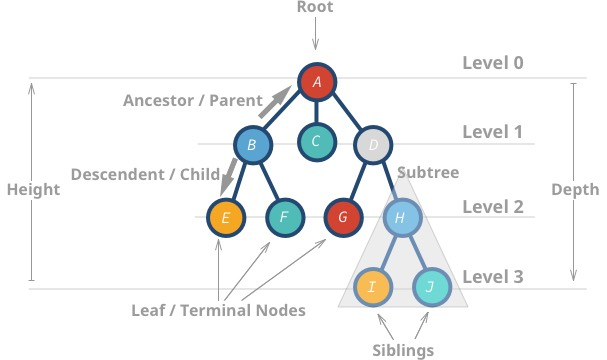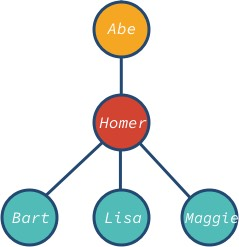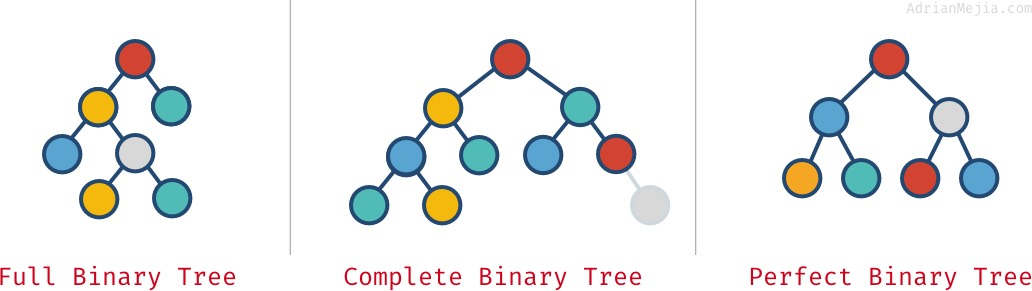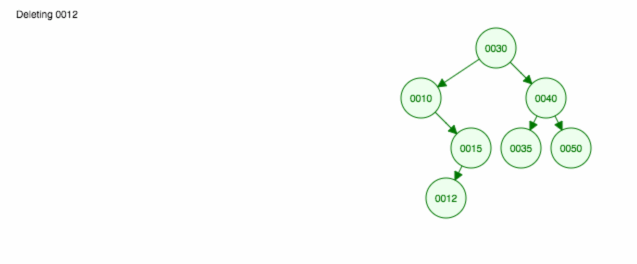Tree data structures are the base for other data structure like Maps and Sets. Also, used on databases performed quick searches. The HTML DOM uses a tree data structure to represents the hierachy of elements. These are some to name a few. In this post, we are going to explore the different types of trees like a binary tree, binary search trees and how to implement them.

In the previous post, we explored the Graph data structures which are a generalized case of trees. Let’s get started learning what tree data structures are!

This post is part of a tutorial series:

Learning Data Structures and Algorithms (DSA) for Beginners

# Trees: basic concepts

A tree is a data structure where a node can zero or more children. Each node contains a value. Like graphs, the connection between nodes is called edges. A tree is a type of graph, but not all graphs are trees (only the acyclic undirected graph are trees).

They are called “trees” because the data structure resembles a tree 🌳. It starts with a root node and branch off with its descendants, and finally, there are leaves.Here are some properties of trees:

• The top-most node is called root.
• A node without children is called leaf node or terminal node.
• Height (h) of the tree is the distance (edge count) between the farthest leaf to the root.
• A has a height of 3
• I has a height of 0
• Depth or level of a node is the distance between the root and the node in question.
• H has a depth of 2
• B has a depth of 1

## Implementing a simple tree data structure

As we saw earlier, a tree node is just a data structure that has a value and has links to their descendants.

Here’s an example of a tree node:

We can create a tree with 3 descendents as follows:

That’s all; we have a tree data structure!The node abe is the root and bart, lisa and maggie are the leaf nodes of the tree. Notice that tree’s node can have a different number of descendants: 0, 1, 3 or any number.

# Binary Trees

Trees nodes can have zero or more children. However, when a tree has at the most 2 children, then it’s called binary tree.

A binary tree is one of the most common forms of trees and has many applications such as:

• Maps
• Sets
• Databases
• Priority Queues
• Querying an LDAP (Lightweight Directory Access Protocol)
• Searching through an XML/HTML file using the Document Object Model (DOM) interface.

## Full, Complete and Perfect binary trees

Depending on how nodes are arranged in a binary tree, it can be full, complete and perfect:

• Full binary tree: each node has exactly 0 or 2 children (but never 1).
• Complete binary tree: when all levels except the last one are full with nodes.
• Perfect binary tree: when all the levels (including the last one) are full of nodes.

Look at this examples:These properties are not always mutually exclusive. You can have more than one:

• A perfect tree is always complete and full.
• Perfect binary trees have precisely 2^k - 1 nodes, where k is the last level of the tree (starting with 1).
• A complete tree is not always full.
• Like in our “complete” example, since it has a parent with only one child. If we remove the rightmost gray node, then we would have a complete and full tree but not perfect.
• A full tree is not always complete and perfect.

# Binary Search Tree (BST)

Binary Search Trees or BST for short are a particular application of binary trees. BST has at most two nodes (like all binary trees). However, the values are in such a way that the left children value must be less than the parent and the right children is must be higher.

Duplicates: Some BST doesn’t allow duplicates while others add the duplicate as a right child. Other implementations might keep a count on a case of duplicates (we are going to do this one later).

Let’s implement a Binary Search Tree!

## BST Implementation

BST are very similar to our previous implementation of a tree. However, there are some differences:

• Nodes can have at most only two children: left and right.
• Nodes values has to be ordered as left < parent < right.

Here’s the tree node. Very similar to what we did before, but we added some handy getters and setters for left and right children. Notice that is also keeping a reference to the parent and we update it every time add children.

Ok, so far we can add a left and right child. Now, let’s do the BST class that enforces the left < parent < right rule.

Let’s implementing insertion.

## BST Node Insertion

To insert a node in a binary tree, we do the following:

1. If a tree is empty, the first node becomes the root and you are done.
2. Compare root/parent’s value if it’s higher go right, if it’s lower go left. If it’s the same, then the value already exists so you can increase the duplicate count (multiplicity).
3. Repeat #2 until we found an empty slot to insert the new node.

Let’s do an illustration how to insert 30, 40, 10, 15, 12, 50:We can implement insert as follows:

We are using a helper function called findNodeAndParent. If we found that the node already exists in the tree, then we increase the multiplicity counter. Let’s see how this function is implemented:

findNodeAndParent goes through the tree searching for the value. It starts at the root (line 2) and then goes left or right based on the value (line 10). If the value already exists, it will return the node found and also the parent. In case that the node doesn’t exist, we still return the parent.

## BST Node Deletion

We know how to insert and search for value. Now, we are going to implement the delete operation. It’s a little trickier than inserting, so let’s explain it with the following cases:

Deleting a leaf node (0 children)

We just remove the reference from node’s parent (15) to be null.

Deleting a node with one child.

In this case, we go to the parent (30) and replace child (10), with a child’s child (15).

Deleting a node with two children

We are removing node 40 that has 2 children (35 and 50). We replace the parent’s (30) child (40) with the child’s right child (50). Then we keep the left child (35) in the same place it was before, so we have to make it the left child of 50.

Another way to do it to remove node 40, is to move the left child (35) up and then keep the right child (50) where it was.

Either way is ok as long as you keep the binary search tree property: left < parent < right.

Deleting the root.

Deleting the root is very similar to deleting nodes with 0, 1, or 2 children that we discussed earlier. The only difference is that afterward, we need to update the reference of the root of the tree.

Here’s an animation of what we discussed.In the animation, it moves up the left child/subtree and keeps the right child/subtree in place.

Now that we have a good idea how it should work, let’s implement it:

Here are some highlights of the implementation:

• First, we search if the node exists. If it doesn’t, we return false and we are done!
• If the node to remove exists, then combine left and right children into one subtree.
• Replace node to delete with the combined subtree.

The function that combines left into right subtree is the following:

For instance, let’s say that we want to combine the following tree and we are about to delete node 30. We would like to combine 30’s left subtree into the right one. The result is this:

Now, and if we make the new subtree the root, then node 30 is no more!

# Binary Tree Transversal

There are different ways of traversing a Binary Tree depending on the order that the nodes are visited: in-order, pre-order and post-order. Also, we can use the DFS and BFS that we learned from the graph post. Let’s go through each one.

In-Order Traversal

In-order traversal visit nodes on this order: left, parent, right.

Let’s use this tree to make the example:

In-order traversal would print out the following values: 3, 4, 5, 10, 15, 30, 40. If the tree is a BST, then the values will be sorted in ascendent order as in our example.

Post-Order Traversal

Post-order traversal visit nodes on this order: left, right, parent.

Post-order traversal would print out the following values: 3, 4, 5, 15, 40, 30, 10.

Pre-Order Traversal and DFS

In-order traversal visit nodes on this order: parent, left, right.

Pre-order traversal would print out the following values: 10, 5, 4, 3, 30, 15, 40. This order of numbers is the same result that we would get if we run the Depth-First Search (DFS).

If you need a refresher on DFS, we covered in details on Graph post.

Breadth-First Search (BFS)

Similar to DFS, we can implement a BFS by switching the Stack by a Queue:

The BFS order is: 10, 5, 30, 4, 15, 40, 3

# Balanced vs. Non-balanced Trees

So far, we have discussed how to add, remove and find elements. However, we haven’t talked about the run times. Let’s think about the worst-case scenarios.

Let’s say that we want to add numbers in ascending order.We will end up with all the nodes on the left side! This unbalanced tree is no better than a LinkedList so finding an element would take O(n). 😱

Looking for something in an unbalanced tree is like looking for a word in the dictionary page by page. When the tree is balanced, you can open the dictionary in the middle and from there you know if you have to go left or right depending on the alphabet and the word you are looking for.

We need to find a way to balance the tree!

If the tree was balanced, then we could find elements in O(log n) instead of going through each node. Let’s talk about what balanced tree means.If we are searching for 7 in the non-balanced tree, we have to go from 1 to 7. However, in the balanced tree, we visit: 4, 6, and 7. It gets even worse with larger trees. If you have one million nodes, searching for a non-existing element might require to visit all million while on a balanced tree it just requires 20 visits! That’s a huge difference!

We are going to solve this issue in the next post using self-balanced trees (AVL trees).

# Summary

We have covered much ground for trees. Let’s sum it up with bullets:

• The tree is a data structure where a node has 0 or more descendants/children.
• Tree nodes don’t have cycles (acyclic). If it has cycles it is a Graph data structure instead.
• Trees with 2 children or less are called: Binary Tree
• When a Binary Tree is sorted in a way that the left value is less than the parent and the right children is higher, then and only then we have a Binary Search Tree.
• You can visit a tree in a pre/post/in-order fashion.
• An unbalanced has a time complexity of O(n). 🤦🏻‍
• A balanced has a time complexity of O(log n). 🎉